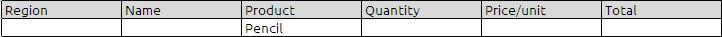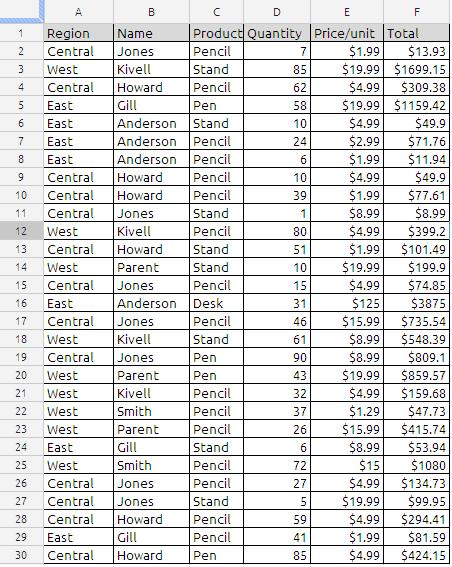# SUMIF FUNCTION

The SUMIF function returns the sum of a collection of numbers, including only numbers that satisfy a specified condition.

SUMIF(range, criteria, sum_range)

range:  The collection containing the values to be tested. range-values is a collection containing any value type.

criteria:  An expression that results in a logical TRUE or FALSE. criteria is an expression that can contain anything as long as the result from comparing criteria to a value in range can be expressed as a Boolean value of TRUE or FALSE.

sum-range:  An optional collection containing the numbers to be summed. Sumrange is a collection containing number, date/time, or duration values. It should have the same dimensions as range.

Notes:
Although range can contain any type of value, it should usually contain values all of the same type. If the sum_range is partially entered then the range will be taken as sum-range

Important 1:
Criteria has to be in quotes unless you put criteria  in  a cell  and refer to it with a cell reference.

Important 2: Criteria for greater than 10 could be :
1)”>10”
2)”>”&A1 where 10 was in cell A1
3) A1 where >10 was in cell A1

Example:
In the sample data if we want to sum total value for the criteria “Pensil “

Formula will be
=sumif(C2:C30,”Pencil”,F2:F30)
To make more generalized formula we can also write as
=sumif(C2:C,”Pencil”,F2:F)
or
=sumif(C2:C30,”Pencil”,F2)
In the third argument (sum-range) if we ignore the whole range still function will works and the function will treat sum-range as range values

other way for achieving the same results for the above stated criteria
Using Sum Function
traditional way of writing with if function
=ArrayFormula(sum(if(C2:C30=”Pencil”,(F2:F30))))
or
=ArrayFormula(SUM((C2:C30=”Pencil”)*(F2:F30)))

Using Sumproduct Function
=ArrayFormula(SUMPRODUCT((C2:C30=”Pencil”)*(F2:F30)))

Using Filter function
=sum(filter(F2:F,C2:C=”Pencil”))

Using Query function
=sum(query(A:F,”select F where C = ‘Pencil'”)

Using DSUM Function
=dsum(A1:F30,”Total”,K2:P3)
where K2:P3 will as below### 10 Responses to SUMIF FUNCTION

1.Yogi Anand says:

Hi Anand:

Great post … you should also, however, add the very versatile QUERY function to the discussion about use of SUMIF, SUM, SUMPRODUCT, FILTER, and DSUM functions.

Cheers!
Yogi
Cloud Computing — Google Docs Way
yogi–anand-consulting.blogspot.com

•anandexcels says:

Dear Yogi,

As Suggested i will do that

2.Christie Hoegerl says:

Very nice pattern and superb subject matter, absolutely nothing else we require :D.

•anandexcels says:

Dear Christie Hoegerl,

You are welcome. Thanks for commenting on my Blog.

anand varma

3.get auto insurance online says:

I like this weblog it’s a master piece! Glad I observed this on google.

4.working capital definition says:

I’ve gone ahead and bookmarked https://anandexcels.wordpress.com/2012/01/28/sumif-function at Digg.com so my friends can see it too.Â I simply used SUMIF FUNCTION anandexcels as the entry title in my Digg.com bookmark, as I figured if it is good enough for you to title your blog post that, then you probably would like to see it bookmarked the same way.

5.Roy Ludera says:

This is a great blog. thanks so much for all the information!

6.Click This Link says:

I’ve gone ahead and bookmarked https://anandexcels.wordpress.com/2012/01/28/sumif-function at Digg.com so my friends can see it too.Â I simply used SUMIF FUNCTION anandexcels as the entry title in my Digg.com bookmark, as I figured if it is good enough for you to title your blog post that, then you probably would like to see it bookmarked the same way.

7.Austin "TheFrosty" Passy says:

Awesome, was looking for something like this. It surely does help a lot!

8.vlookup says:

Learn vlookup , excel hlookup , sumif , vba etc. We are excel consultant and access programmer with expertise in advanced Excel formulas, modeling, macros, and VBA.